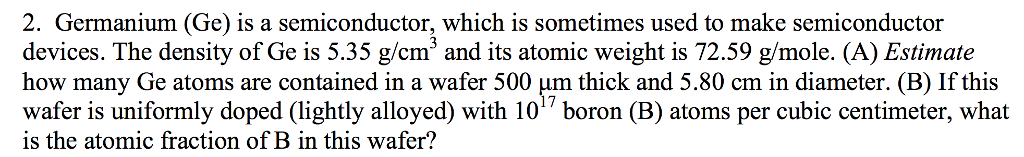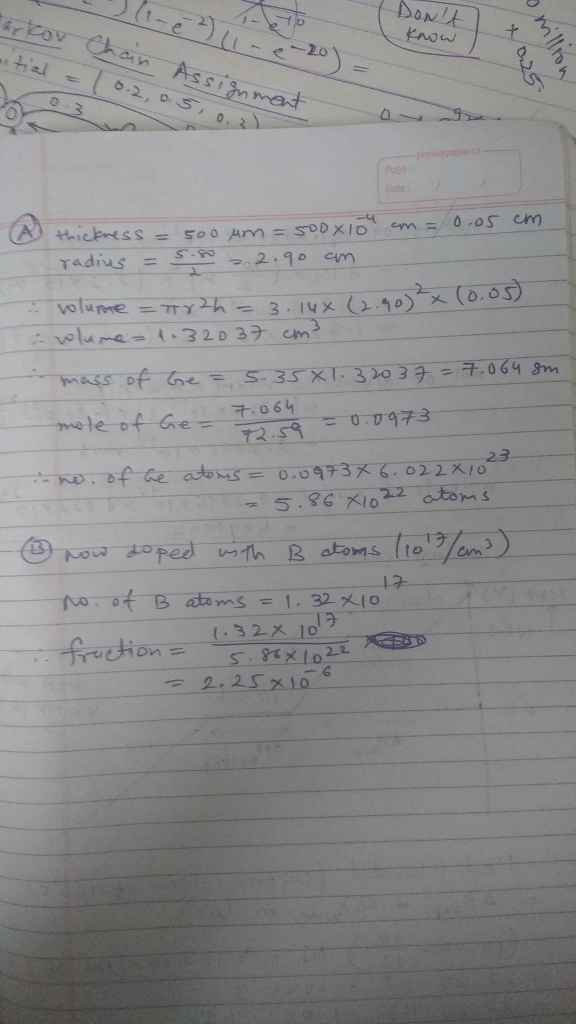# Question & Answer: Germanium (Ge) is a semiconductor, which is sometimes used to make semiconductor…..Germanium (Ge) is a semiconductor, which is sometimes used to make semiconductor devices. The density of Ge is 5.35 g/cm^3 and its atomic weight is 72.59 g/mole. (A) Estimate how many Ge atoms are contained in a wafer 500 mu m thick and 5.80 cm in diameter. (B) If this wafer is uniformly doped (lightly alloyed) with 10^17 boron (B) atoms per cubic centimeter, what is the atomic fraction of B in this wafer?